## How to Create Data Visualization for Association Rules in Data Mining

Association rule learning is used in machine learning for discovering interesting relations between variables. Apriori algorithm is a popular algorithm for association rules mining and extracting frequent itemsets with applications in association rule learning. It has been designed to operate on databases containing transactions, such as purchases by customers of a store (market basket analysis).  Besides market basket analysis this algorithm can be applied to other problems. For example in web user navigation domain we can search for rules like customer who visited web page A and page B also visited page C.

Python sklearn library does not have Apriori algorithm but recently I come across post  where python library MLxtend was used for Market Basket Analysis. MLxtend has modules for different tasks. In this post I will share how to create data visualization for association rules in data mining using MLxtend for getting association rules and NetworkX module for charting the diagram. First we need to get association rules.

### Getting Association Rules from Array Data

To get association rules you can run the following code

dataset = [['Milk', 'Onion', 'Nutmeg', 'Kidney Beans', 'Eggs', 'Yogurt'],
['Dill', 'Onion', 'Nutmeg', 'Kidney Beans', 'Eggs', 'Yogurt'],
['Milk', 'Apple', 'Kidney Beans', 'Eggs'],
['Milk', 'Unicorn', 'Corn', 'Kidney Beans', 'Yogurt'],
['Corn', 'Onion', 'Onion', 'Kidney Beans', 'Ice cream', 'Eggs']]

import pandas as pd
from mlxtend.preprocessing import OnehotTransactions
from mlxtend.frequent_patterns import apriori

oht = OnehotTransactions()
oht_ary = oht.fit(dataset).transform(dataset)
df = pd.DataFrame(oht_ary, columns=oht.columns_)
print (df)

frequent_itemsets = apriori(df, min_support=0.6, use_colnames=True)
print (frequent_itemsets)

association_rules(frequent_itemsets, metric="confidence", min_threshold=0.7)
rules = association_rules(frequent_itemsets, metric="lift", min_threshold=1.2)
print (rules)

"""
Below is the output
support                     itemsets
0       0.8                       [Eggs]
1       1.0               [Kidney Beans]
2       0.6                       [Milk]
3       0.6                      [Onion]
4       0.6                     [Yogurt]
5       0.8         [Eggs, Kidney Beans]
6       0.6                [Eggs, Onion]
7       0.6         [Kidney Beans, Milk]
8       0.6        [Kidney Beans, Onion]
9       0.6       [Kidney Beans, Yogurt]
10      0.6  [Eggs, Kidney Beans, Onion]

antecedants            consequents  support  confidence  lift
0  (Kidney Beans, Onion)                 (Eggs)      0.6        1.00  1.25
1   (Kidney Beans, Eggs)                (Onion)      0.8        0.75  1.25
2                (Onion)   (Kidney Beans, Eggs)      0.6        1.00  1.25
3                 (Eggs)  (Kidney Beans, Onion)      0.8        0.75  1.25
4                (Onion)                 (Eggs)      0.6        1.00  1.25
5                 (Eggs)                (Onion)      0.8        0.75  1.25

"""


### Confidence and Support in Data Mining

To select interesting rules we can use best-known constraints which are a minimum thresholds on confidence and support.
Support is an indication of how frequently the itemset appears in the dataset.
Confidence is an indication of how often the rule has been found to be true. 

support=rules.as_matrix(columns=['support'])
confidence=rules.as_matrix(columns=['confidence'])


Below is the scatter plot for support and confidence:Association rules – scatter plot

And here is the python code to build scatter plot. Since few points here have the same values I added small random values to show all points.

import random
import matplotlib.pyplot as plt

for i in range (len(support)):
support[i] = support[i] + 0.0025 * (random.randint(1,10) - 5)
confidence[i] = confidence[i] + 0.0025 * (random.randint(1,10) - 5)

plt.scatter(support, confidence,   alpha=0.5, marker="*")
plt.xlabel('support')
plt.ylabel('confidence')
plt.show()


### How to Create Data Visualization with NetworkX for Association Rules in Data Mining

To represent association rules as diagram, NetworkX python library is utilized in this post. Here is the association rule example :
(Kidney Beans, Onion) ==> (Eggs)

Directed graph below is built for this rule and shown below. Arrows are drawn as just thicker blue stubs. The node with R0 identifies one rule, and it will have always incoming and outcoming edges. Incoming edge(s) will represent antecedants and the stub (arrow) will be next to node.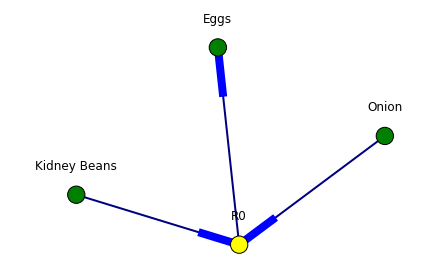Below is the example of graph for all rules extracted from example dataset.Here is the source code to build association rules with NetworkX. To call function use draw_graph(rules, 6)

def draw_graph(rules, rules_to_show):
import networkx as nx
G1 = nx.DiGraph()

color_map=[]
N = 50
colors = np.random.rand(N)
strs=['R0', 'R1', 'R2', 'R3', 'R4', 'R5', 'R6', 'R7', 'R8', 'R9', 'R10', 'R11']

for i in range (rules_to_show):
G1.add_nodes_from(["R"+str(i)])

for a in rules.iloc[i]['antecedants']:

G1.add_nodes_from([a])

G1.add_edge(a, "R"+str(i), color=colors[i] , weight = 2)

for c in rules.iloc[i]['consequents']:

G1.add_nodes_from()

G1.add_edge("R"+str(i), c, color=colors[i],  weight=2)

for node in G1:
found_a_string = False
for item in strs:
if node==item:
found_a_string = True
if found_a_string:
color_map.append('yellow')
else:
color_map.append('green')

edges = G1.edges()
colors = [G1[u][v]['color'] for u,v in edges]
weights = [G1[u][v]['weight'] for u,v in edges]

pos = nx.spring_layout(G1, k=16, scale=1)
nx.draw(G1, pos, edges=edges, node_color = color_map, edge_color=colors, width=weights, font_size=16, with_labels=False)

for p in pos:  # raise text positions
pos[p] += 0.07
nx.draw_networkx_labels(G1, pos)
plt.show()


### Data Visualization for Online Retail Data Set

To get real feeling and testing on visualization we can take available online retail store dataset and apply the code for association rules graph. For downloading retail data and formatting some columns the code from  was used.

Below are the result of scatter plot for support and confidence. To build the scatter plot seaborn library was used this time. Also you can find below visualization for association rules (first 10 rules) for retail data set.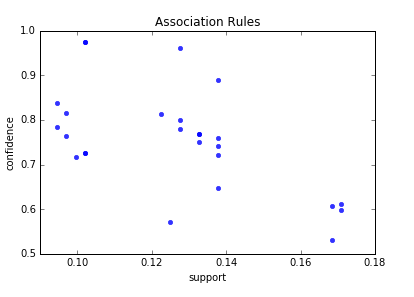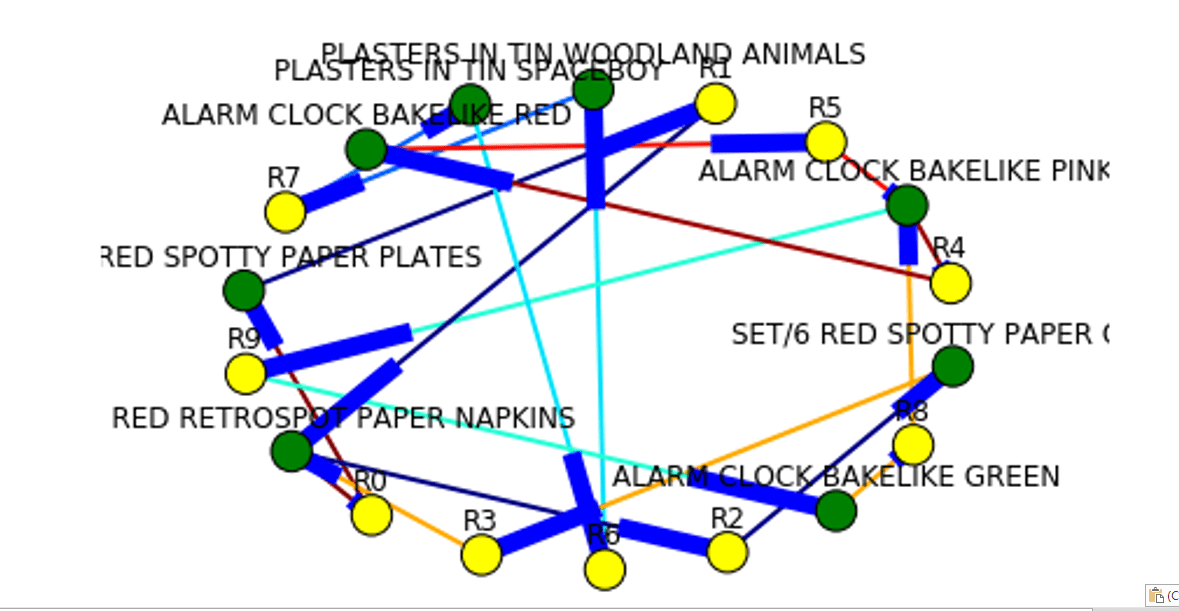Here is the python full source code for data visualization association rules in data mining.



dataset = [['Milk', 'Onion', 'Nutmeg', 'Kidney Beans', 'Eggs', 'Yogurt'],
['Dill', 'Onion', 'Nutmeg', 'Kidney Beans', 'Eggs', 'Yogurt'],
['Milk', 'Apple', 'Kidney Beans', 'Eggs'],
['Milk', 'Unicorn', 'Corn', 'Kidney Beans', 'Yogurt'],
['Corn', 'Onion', 'Onion', 'Kidney Beans', 'Ice cream', 'Eggs']]

import pandas as pd
from mlxtend.preprocessing import OnehotTransactions
from mlxtend.frequent_patterns import apriori

oht = OnehotTransactions()
oht_ary = oht.fit(dataset).transform(dataset)
df = pd.DataFrame(oht_ary, columns=oht.columns_)
print (df)

frequent_itemsets = apriori(df, min_support=0.6, use_colnames=True)
print (frequent_itemsets)

from mlxtend.frequent_patterns import association_rules

association_rules(frequent_itemsets, metric="confidence", min_threshold=0.7)
rules = association_rules(frequent_itemsets, metric="lift", min_threshold=1.2)
print (rules)

support=rules.as_matrix(columns=['support'])
confidence=rules.as_matrix(columns=['confidence'])

import random
import matplotlib.pyplot as plt

for i in range (len(support)):
support[i] = support[i] + 0.0025 * (random.randint(1,10) - 5)
confidence[i] = confidence[i] + 0.0025 * (random.randint(1,10) - 5)

plt.scatter(support, confidence,   alpha=0.5, marker="*")
plt.xlabel('support')
plt.ylabel('confidence')
plt.show()

import numpy as np

def draw_graph(rules, rules_to_show):
import networkx as nx
G1 = nx.DiGraph()

color_map=[]
N = 50
colors = np.random.rand(N)
strs=['R0', 'R1', 'R2', 'R3', 'R4', 'R5', 'R6', 'R7', 'R8', 'R9', 'R10', 'R11']

for i in range (rules_to_show):
G1.add_nodes_from(["R"+str(i)])

for a in rules.iloc[i]['antecedants']:

G1.add_nodes_from([a])

G1.add_edge(a, "R"+str(i), color=colors[i] , weight = 2)

for c in rules.iloc[i]['consequents']:

G1.add_nodes_from()

G1.add_edge("R"+str(i), c, color=colors[i],  weight=2)

for node in G1:
found_a_string = False
for item in strs:
if node==item:
found_a_string = True
if found_a_string:
color_map.append('yellow')
else:
color_map.append('green')

edges = G1.edges()
colors = [G1[u][v]['color'] for u,v in edges]
weights = [G1[u][v]['weight'] for u,v in edges]

pos = nx.spring_layout(G1, k=16, scale=1)
nx.draw(G1, pos, edges=edges, node_color = color_map, edge_color=colors, width=weights, font_size=16, with_labels=False)

for p in pos:  # raise text positions
pos[p] += 0.07
nx.draw_networkx_labels(G1, pos)
plt.show()

draw_graph (rules, 6)

df = pd.read_excel('http://archive.ics.uci.edu/ml/machine-learning-databases/00352/Online%20Retail.xlsx')

df['Description'] = df['Description'].str.strip()
df.dropna(axis=0, subset=['InvoiceNo'], inplace=True)
df['InvoiceNo'] = df['InvoiceNo'].astype('str')
df = df[~df['InvoiceNo'].str.contains('C')]

basket = (df[df['Country'] =="France"]
.groupby(['InvoiceNo', 'Description'])['Quantity']
.sum().unstack().reset_index().fillna(0)
.set_index('InvoiceNo'))

def encode_units(x):
if x <= 0:
return 0
if x >= 1:
return 1

basket_sets = basket.applymap(encode_units)
basket_sets.drop('POSTAGE', inplace=True, axis=1)

frequent_itemsets = apriori(basket_sets, min_support=0.07, use_colnames=True)

rules = association_rules(frequent_itemsets, metric="lift", min_threshold=1)
rules.head()

print (rules)

support=rules.as_matrix(columns=['support'])
confidence=rules.as_matrix(columns=['confidence'])

import seaborn as sns1

for i in range (len(support)):
support[i] = support[i]
confidence[i] = confidence[i]

plt.title('Association Rules')
plt.xlabel('support')
plt.ylabel('confidence')
sns1.regplot(x=support, y=confidence, fit_reg=False)

plt.gcf().clear()
draw_graph (rules, 10)



References

## Regression and Classification Decision Trees – Building with Python and Running Online

According to survey  Decision Trees constitute one of the 10 most popular data mining algorithms.
Decision trees used in data mining are of two main types:
Classification tree analysis is when the predicted outcome is the class to which the data belongs.
Regression tree analysis is when the predicted outcome can be considered a real number (e.g. the price of a house, or a patient’s length of stay in a hospital).

In the previous posts I already covered how to create Regression Decision Trees with python:

In this post you will find more simplified python code for classification and regression decision trees. Online link to run decision tree also will be provided. This is very useful if you want see results immediately without coding.

To run the code provided here you need just change file path to file containing data. The Decision Trees in this post are tested on simple artificial dataset that was motivated by doing feature selection for blog data:

Getting Data-Driven Insights from Blog Data Analysis with Feature Selection

Dataset
Our dataset consists of 3 columns in csv file and shown below. It has 2 independent variables (features or X columns) – categorical and numerical, and dependent numerical variable (target or Y column). The script is assuming that the target column is the last column. Below is the dataset that is used in this post:


X1	X2	Y
red	1	100
red	2	99
red	1	85
red	2	100
red	1	79
red	2	100
red	1	100
red	1	85
red	2	100
red	1	79
blue	2	22
blue	1	20
blue	2	21
blue	1	13
blue	2	10
blue	1	22
blue	2	20
blue	1	21
blue	2	13
blue	1	10
blue	1	22
blue	2	20
blue	1	21
blue	2	13
blue	1	10
blue	2	22
blue	1	20
blue	2	21
blue	1	13
green	2	10
green	1	22
green	2	20
green	1	21
green	2	13
green	1	10
green	2	22
green	1	20
green	1	13
green	2	22
green	1	20
green	2	21
green	1	13
green	2	10


You can use dataset with different number of columns for independent variables without changing the code.

For converting categorical variable to numerical we use here pd.get_dummies(dataframe) method from pandas library. Here dataframe is our input data. So the column with “green”, “red”, “yellow” will be transformed in 3 columns with 0,1 values in each (one hot encoding scheme). Below are the few first rows after converting:


N   X2  X1_blue  X1_green  X1_red    Y
0    1      0.0       0.0     1.0  100
1    2      0.0       0.0     1.0   99
2    1      0.0       0.0     1.0   85
3    2      0.0       0.0     1.0  100


Python Code
Two scripts are provided here – regressor and classifier. For classifier the target variable should be categorical. We use however same dataset but convert numerical continuous variable to classes with labels (A,B,C) within the script based on inputted bin ranges ([15,50,100] which means bins 0-15, 15.001-50, 50.001-100). We use this after applying get_dummies

What if you have categorical target? Calling get_dummies will convert it to numerical too but we do not want this. In this case you need specify explicitly what columns need to be converted via parameter columns As per the documentation:
columns : list-like, default None. This is column names in the DataFrame to be encoded. If columns is None then all the columns with object or category dtype will be converted. 
In our example we would need to do specify column X1 like this:
dataframe=pd.get_dummies(dataframe, columns=[“X1”])

The results of running scripts are decision trees shown below:
Decision Tree RegressionDecision Tree Classification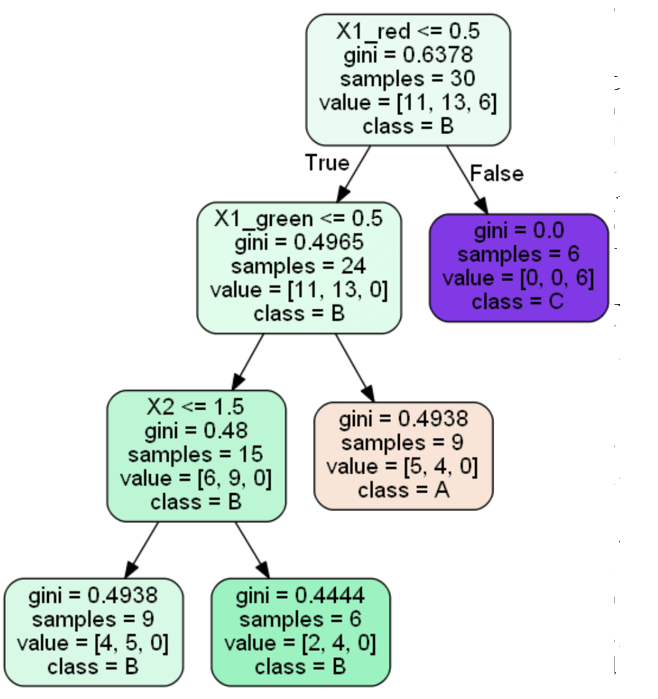Running Decision Trees Online
In case you do not want to play with python code, you can run Decision Tree algorithms online at ML Sandbox
All that you need is just enter data into the data fields, here are the instructions:

1. Go to ML Sandbox
2. Select Decision Classifier OR Decision Regressor
3. Enter data (first row should have headers) OR click “Load Default Values” to load the example data from this post. See screenshot below
4. Click “Run Now“.
5. Click “View Run Results
6. If you do not see yet data wait for a minute or so and click “Refresh Page” and you will see results
7. Note: your dependent variable (target variable or Y variable) should be in most right column. Also do not use space in the words (header and data)

Conclusion
Decision Trees belong to the top 10 machine learning or data mining algorithms and in this post we looked how to build Decision Trees with python. The source code provided is the end of this post. We looked also how do this if one or more columns are categorical. The source code was tested on simple categorical and numerical example and provided in this post. Alternatively you can run same algorithm online at ML Sandbox

References

Here is the python computer code of the scripts.
DecisionTreeRegressor


# -*- coding: utf-8 -*python computer code-

import pandas as pd
from sklearn.cross_validation import train_test_split
from sklearn.tree import DecisionTreeRegressor

import subprocess

from sklearn.tree import  export_graphviz

def visualize_tree(tree, feature_names):

with open("dt.dot", 'w') as f:

export_graphviz(tree, out_file=f, feature_names=feature_names,  filled=True, rounded=True )

command = ["C:\\Program Files (x86)\\Graphviz2.38\\bin\\dot.exe", "-Tpng", "C:\\Users\\Owner\\Desktop\\A\\Python_2016_A\\dt.dot", "-o", "dt.png"]

try:
subprocess.check_call(command)
except:
exit("Could not run dot, ie graphviz, to "
"produce visualization")

filename = "C:\\Users\\Owner\\Desktop\\A\\Blog Analytics\\data1.csv"
dataframe = pd.read_csv(filename, sep= ',' )

cols = dataframe.columns.tolist()
last_col_header = cols[-1]

dataframe=pd.get_dummies(dataframe)
cols = dataframe.columns.tolist()

cols.insert(len(dataframe.columns)-1, cols.pop(cols.index(last_col_header)))
dataframe = dataframe.reindex(columns= cols)

print (dataframe)

array = dataframe.values
X = array[:,0:len(dataframe.columns)-1]
Y = array[:,len(dataframe.columns)-1]
print ("--X----")
print (X)
print ("--Y----")
print (Y)

X_train, X_test, y_train, y_test = train_test_split( X, Y, test_size = 0.3, random_state = 100)

clf = DecisionTreeRegressor( random_state = 100,
max_depth=3, min_samples_leaf=4)
clf.fit(X_train, y_train)

visualize_tree(clf, dataframe.columns)


DecisionTreeClassifier


# -*- coding: utf-8 -*-

import pandas as pd
from sklearn.cross_validation import train_test_split
from sklearn.tree import DecisionTreeClassifier

import subprocess

from sklearn.tree import  export_graphviz

def visualize_tree(tree, feature_names, class_names):

with open("dt.dot", 'w') as f:

export_graphviz(tree, out_file=f, feature_names=feature_names,  filled=True, rounded=True, class_names=class_names )

command = ["C:\\Program Files (x86)\\Graphviz2.38\\bin\\dot.exe", "-Tpng", "C:\\Users\\Owner\\Desktop\\A\\Python_2016_A\\dt.dot", "-o", "dt.png"]

try:
subprocess.check_call(command)
except:
exit("Could not run dot, ie graphviz, to "
"produce visualization")

values=[15,50,100]
def convert_to_label (a):
count=0
for v in values:
if (a <= v) :
return chr(ord('A') + count)
else:
count=count+1

filename = "C:\\Users\\Owner\\Desktop\\A\\Blog Analytics\\data1.csv"
dataframe = pd.read_csv(filename, sep= ',' )

cols = dataframe.columns.tolist()
last_col_header = cols[-1]
dataframe=pd.get_dummies(dataframe)
cols = dataframe.columns.tolist()

print (dataframe)

for index, row in dataframe.iterrows():
dataframe.loc[index, "Y"] = convert_to_label(dataframe.loc[index, "Y"])

cols.insert(len(dataframe.columns)-1, cols.pop(cols.index('Y')))
dataframe = dataframe.reindex(columns= cols)

print (dataframe)

array = dataframe.values
X = array[:,0:len(dataframe.columns)-1]
Y = array[:,len(dataframe.columns)-1]
print ("--X----")
print (X)
print ("--Y----")
print (Y)

X_train, X_test, y_train, y_test = train_test_split( X, Y, test_size = 0.3, random_state = 100)

clf = DecisionTreeClassifier(criterion = "gini", random_state = 100,
max_depth=3, min_samples_leaf=4)

clf.fit(X_train, y_train)

clm=dataframe[last_col_header]
clmvalues = clm.unique()
visualize_tree(clf, dataframe.columns, clmvalues )


## Data Visualization of Word Correlations with NetworkX

This is a continuation of my previous post, found here Combining Machine Learning and Data Scraping. Data visualization is added to show correlations between words. The graph was built using NetworkX python library.
The input for the graph is the array corr_data with 3 columns : pair of words and correlation between them. This was calculated in the previous post.

In this post are added two functions:
build_graph_for_all – it is taking words from matrix for the first N rows and adding to the graph.
The graph is shown below.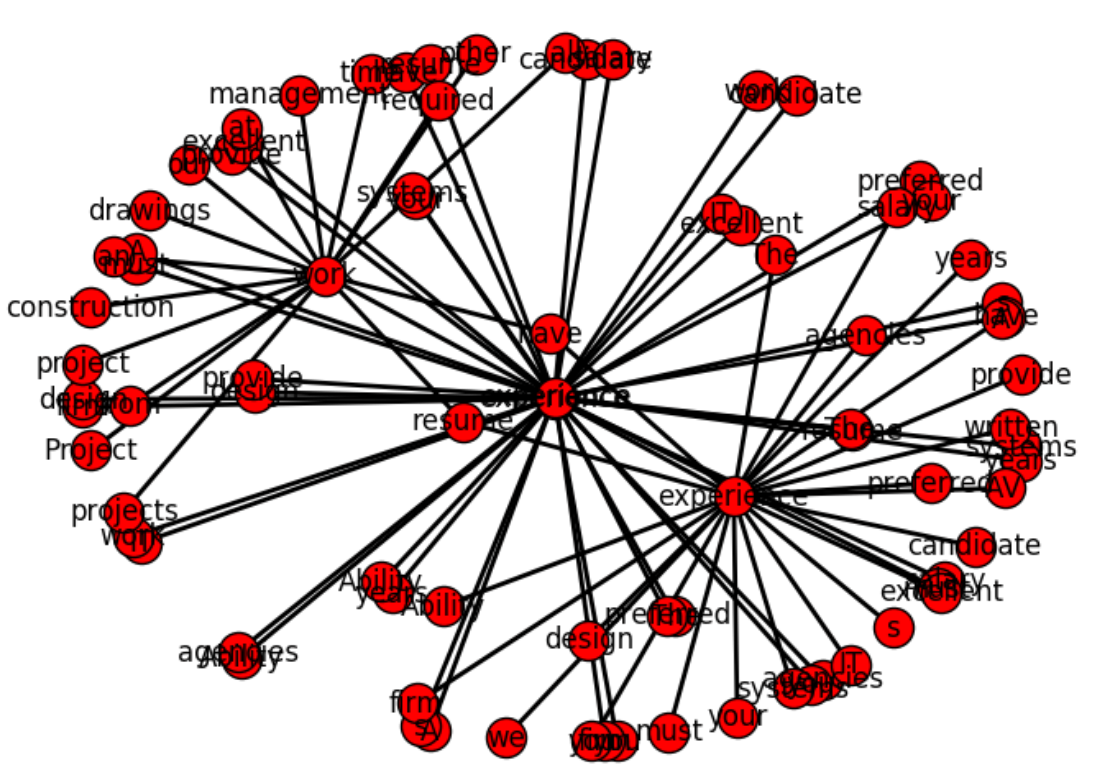The Second function build_graph is taking specific word and adding to graph only edge that have this word. The process is repeating but now it is adding edges to other words on the graph. This is recursive function. Below in the python code are shown these functions.

Python computer code:


import networkx as nx
import matplotlib.pyplot as plt
G=nx.Graph()

existing_edges = {}

def build_graph(w, lev):
if (lev > 5)  :
return
for z in corr_data:
ind=-1
if z == w:
ind=0
ind1=1
if z == w:
ind ==1
ind1 =0

if ind == 0 or ind == 1:
if  str(w) + "_" + str(corr_data[ind1]) not in existing_edges :

G.add_node(str(corr_data[ind]))
existing_edges[str(w) + "_" + str(corr_data[ind1])] = 1;
G.add_edge(w,str(corr_data[ind1]))

build_graph(corr_data[ind1], lev+1)

existing_nodes = {}
def build_graph_for_all():
count=0
for d in corr_data:
if (count > 40) :
return
if  d not in existing_edges :
G.add_node(str(d))
if  d not in existing_edges :
G.add_node(str(d))
G.add_edge(str(d), str(d))
count=count + 1

build_graph_for_all()

print (G.nodes(data=True))
plt.show()
nx.draw(G, width=2, with_labels=True)
plt.savefig("path1.png")

w="design"
G.add_node(w)
build_graph(w, 0)

print (G.nodes(data=True))
plt.show()
nx.draw(G, width=2, with_labels=True)
plt.savefig("path.png")


In this post we created script that can be used to draw plot of connections between the words. In the near future I am planning to apply this technique to real problem. Below is the full source code.


# -*- coding: utf-8 -*-

import numpy as np
import nltk
import csv
import re
from scipy.stats.stats import pearsonr

def remove_html_tags(text):
"""Remove html tags from a string"""
clean = re.compile('<.*?>')
return re.sub(clean, '', text)

fn="C:\\Users\\Owner\\Desktop\\A\\Scrapping\\craigslist\\result-jobs-multi-pages-content.csv"

docs=[]
def load_file(fn):
start=1
file_urls=[]

strtext=""
with open(fn, encoding="utf8" ) as f:
csv_f = csv.reader(f)
for i, row in enumerate(csv_f):
if i >=  start  :
file_urls.append (row)

strtext=strtext + replaceNotNeeded(str(stripNonAlphaNum(row)))
docs.append (str(stripNonAlphaNum(row)))

return strtext

# Given a text string, remove all non-alphanumeric
# characters (using Unicode definition of alphanumeric).

def stripNonAlphaNum(text):
import re
return re.compile(r'\W+', re.UNICODE).split(text)

def replaceNotNeeded(text):
text=text.replace("'","").replace(",","").replace ("''","").replace("'',","")
text=text.replace(" and ", " ").replace (" to ", " ").replace(" a "," ").replace(" the "," ").replace(" of "," ").replace(" in "," ").replace(" for ", " ").replace(" or ", " ")
text=text.replace(" will ", " ").replace (" on ", " ").replace(" be "," ").replace(" with "," ").replace(" is "," ").replace(" as "," ")
text=text.replace("    "," ").replace("   "," ").replace("  "," ")
return text

txt=load_file(fn)
print (txt)

tokens = nltk.wordpunct_tokenize(str(txt))

my_count = {}
for word in tokens:
try: my_count[word] += 1
except KeyError: my_count[word] = 1

print (my_count)

data = []

sortedItems = sorted(my_count , key=my_count.get , reverse = True)
item_count=0
for element in sortedItems :
if (my_count.get(element) > 3):
data.append([element, my_count.get(element)])
item_count=item_count+1

N=5
topN = []
corr_data =[]
for z in range(N):
topN.append (data[z])

wcount = [[0 for x in range(500)] for y in range(2000)]
docNumber=0
for doc in docs:

for z in range(item_count):

wcount[docNumber][z] = doc.count (data[z])
docNumber=docNumber+1

print ("calc correlation")

for ii in range(N-1):
for z in range(item_count):

r_row, p_value = pearsonr(np.array(wcount)[:, ii], np.array(wcount)[:, z])
print (r_row, p_value)
if r_row > 0.6 and r_row < 1:
corr_data.append ([topN[ii],  data[z], r_row])

print ("correlation data")
print (corr_data)

import networkx as nx
import matplotlib.pyplot as plt
G=nx.Graph()

existing_edges = {}

def build_graph(w, lev):
if (lev > 5)  :
return
for z in corr_data:
ind=-1
if z == w:
ind=0
ind1=1
if z == w:
ind ==1
ind1 =0

if ind == 0 or ind == 1:
if  str(w) + "_" + str(corr_data[ind1]) not in existing_edges :

G.add_node(str(corr_data[ind]))
existing_edges[str(w) + "_" + str(corr_data[ind1])] = 1;
G.add_edge(w,str(corr_data[ind1]))

build_graph(corr_data[ind1], lev+1)

existing_nodes = {}
def build_graph_for_all():
count=0
for d in corr_data:
if (count > 40) :
return
if  d not in existing_edges :
G.add_node(str(d))
if  d not in existing_edges :
G.add_node(str(d))
G.add_edge(str(d), str(d))
count=count + 1

build_graph_for_all()

print (G.nodes(data=True))
plt.show()
nx.draw(G, width=2, with_labels=True)
plt.savefig("path5.png")

w="design"

G.add_node(w)

build_graph(w, 10)

print (G.nodes(data=True))
plt.show()
nx.draw(G, width=2, with_labels=True)
plt.savefig("path.png")


## Combining Machine Learning and Data Scraping

I often come across web posts about extracting data (data scraping) from websites. For example recently in  Scrapy tool was used for web scraping with Python. Once we get scraping data we can use extracted information in many different ways. As computer algorithms evolve and can do more, the number of cases where machine learning is used to get insights from extracted data is increasing. In the case of extracted data from text, exploring commonly co-occurring terms can give useful information.

In this post we will see the example of such usage including computing of correlation.

Our example is taken from  where job site was scraped and job descriptions were processed further to extract information about requested skills. The job description text was analyzed to explore commonly co-occurring technology-related terms, focusing on frequent skills required by employers.

Data visualization also was performed – the graph was created to show connections between different words (skills) for the few most frequent terms. This looks useful as the user can see related skills for the given term which can be not visible from text ads.

The plot was built based on correlations between words in the text, so it is possible also to visualize the strength of connections between words.

Inspired by this example I built the python script that can calculate correlation and does the following:

• Opens csv file with the text data and load data into memory. (job descriptions are only in one column)
• Counts top N number based on the frequency (N is the number that should be set, for example N=5)
• For each word from the top N words it calculate correlation between this word and all other words.
• The words with correlation more than some threshold (0.4 for example) are saved to array and then printed as pair of words and correlation between them. This is the final output of the script. This result can be used for printing graph of connections between words.

Python function pearsonr was used for calculating correlation. It allows to calculate Pearson correlation coefficient which is a measure of the linear correlation between two variables X and Y. It has a value between +1 and −1, where 1 is total positive linear correlation, 0 is no linear correlation, and −1 is total negative linear correlation. It is widely used in the sciences.

The function pearsonr returns two values: pearson coefficient and the p-value for testing non-correlation. 

The script is shown below.

Thus we saw how data scraping can be used together with machine learning to produce meaningful results.
The created script allows to calculate correlation between terms in the corpus that can be used to draw plot of connections between the words like it was done in .

See how to do web data scraping here with newspaper python module or with beautifulsoup module

Here you can find how to build graph plot


# -*- coding: utf-8 -*-

import numpy as np
import nltk
import csv
import re
from scipy.stats.stats import pearsonr

def remove_html_tags(text):
"""Remove html tags from a string"""
clean = re.compile('<.*?>')
return re.sub(clean, '', text)

fn="C:\\Users\\Owner\\Desktop\\Scrapping\\datafile.csv"

docs=[]
def load_file(fn):
start=1
file_urls=[]

strtext=""
with open(fn, encoding="utf8" ) as f:
csv_f = csv.reader(f)
for i, row in enumerate(csv_f):
if i >=  start  :
file_urls.append (row)

strtext=strtext + str(stripNonAlphaNum(row))
docs.append (str(stripNonAlphaNum(row)))

return strtext

# Given a text string, remove all non-alphanumeric
# characters (using Unicode definition of alphanumeric).

def stripNonAlphaNum(text):
import re
return re.compile(r'\W+', re.UNICODE).split(text)

txt=load_file(fn)
print (txt)

tokens = nltk.wordpunct_tokenize(str(txt))

my_count = {}
for word in tokens:
try: my_count[word] += 1
except KeyError: my_count[word] = 1

data = []

sortedItems = sorted(my_count , key=my_count.get , reverse = True)
item_count=0
for element in sortedItems :
if (my_count.get(element) > 3):
data.append([element, my_count.get(element)])
item_count=item_count+1

N=5
topN = []
corr_data =[]
for z in range(N):
topN.append (data[z])

wcount = [[0 for x in range(500)] for y in range(2000)]
docNumber=0
for doc in docs:

for z in range(item_count):

wcount[docNumber][z] = doc.count (data[z])
docNumber=docNumber+1

print ("calc correlation")

for ii in range(N-1):
for z in range(item_count):

r_row, p_value = pearsonr(np.array(wcount)[:, ii], np.array(wcount)[:, z])
print (r_row, p_value)
if r_row > 0.4 and r_row < 1:
corr_data.append ([topN[ii],  data[z], r_row])

print ("correlation data")
print (corr_data)


## Algorithms, Metrics and Online Tool for Clustering

One of the key techniques of exploratory data mining is clustering – separating instances into distinct groups based on some measure of similarity.  In this post we will review how we can do clustering, evaluate and visualize results using online ML Sandbox tool from this website. This tool allows to run some machine learning algorithms without coding and setup/install. The following components will be explored:

Clustering Algorithms
K-means Clustering Algorithm – is well known algorithm as the idea of this algorithm goes back to 1957.  The algorithm requires to input number of clusters and data. k-means clustering aims to partition n observations into k clusters in which each observation belongs to the cluster with the nearest mean.. Below are shown results of K-means clustering of Iris dataset (only 2 dimensions shown) and clustering result for S1 dataset (see dataset section for more details).k-means clustering Iris dataset
Fig 1. K-means clustering of Iris dataset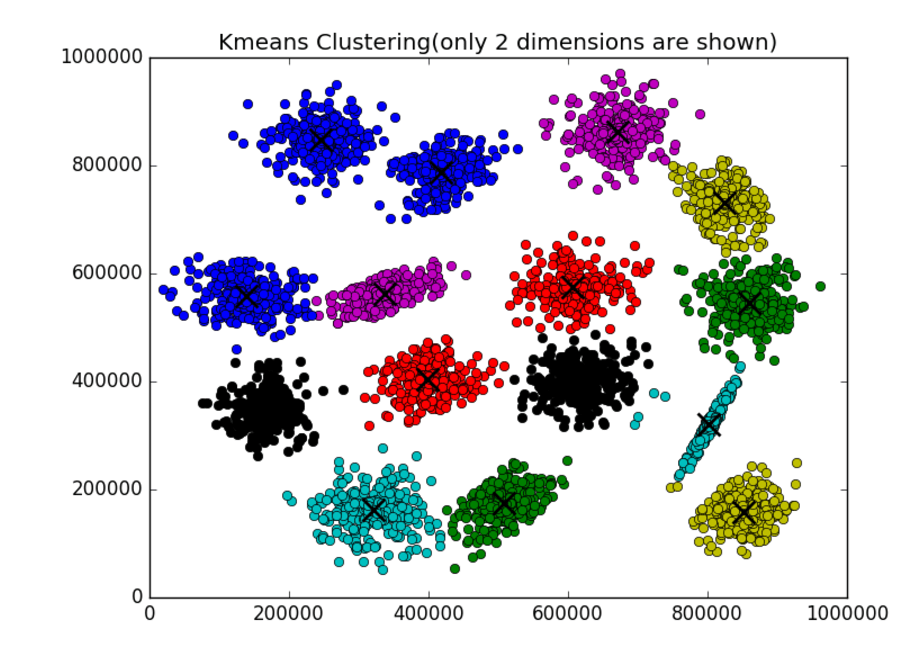Fig 2. K-means clustering of S1 dataset

Affinity Propagation – performs affinity propagation clustering of data. In statistics and data mining, affinity propagation (AP) is a clustering algorithm based on the concept of “message passing” between data points. Unlike clustering algorithms such as k-means or k-medoids, affinity propagation does not require the number of clusters to be determined or estimated before running the algorithm. Similar to k-medoids, affinity propagation finds “exemplars”, members of the input set that are representative of clusters.

Hierarchical clustering (HC) – (also called hierarchical cluster analysis or HCA) is a method of cluster analysis which seeks to build a hierarchy of clusters. Strategies for hierarchical clustering generally fall into two types:

• Agglomerative: This is a “bottom up” approach: each observation starts in its own cluster, and pairs of clusters are merged as one moves up the hierarchy.
• Divisive: This is a “top down” approach: all observations start in one cluster, and splits are performed recursively as one moves down the hierarchy.

In general, the merges and splits are determined in a greedy manner. The results of hierarchical clustering are usually presented in a dendrogram. 

Birch algorithm – Back in the 1990s considerable effort has been put into improving the performance of existing algorithms. Among them is BIRCH (Zhang et al., 1996) 

BIRCH (balanced iterative reducing and clustering using hierarchies) is an unsupervised data mining algorithm used to perform hierarchical clustering over particularly large data-sets. An advantage of BIRCH is its ability to incrementally and dynamically cluster incoming, multi-dimensional metric data points in an attempt to produce the best quality clustering for a given set of resources (memory and time constraints). In most cases, BIRCH only requires a single scan of the database. 

Performance metrics for clustering algorithms

Silhouette refers to a method of interpretation and validation of consistency within clusters of data. The technique provides a succinct graphical representation of how well each object lies within its cluster. It was first described by Peter J. Rousseeuw in 1986.

The silhouette value is a measure of how similar an object is to its own cluster (cohesion) compared to other clusters (separation). The silhouette ranges from -1 to 1, where a high value indicates that the object is well matched to its own cluster and poorly matched to neighboring clusters. If most objects have a high value, then the clustering configuration is appropriate. If many points have a low or negative value, then the clustering configuration may have too many or too few clusters.
The silhouette can be calculated with any distance metric, such as the Euclidean distance or the Manhattan distance.

Here is the python source code how to calculate the silhouette value for k-means clustering


from sklearn import cluster
from sklearn import metrics
import numpy as np

k=2
data = np.array([[1, 2],
[5, 8],
[1.5, 1.8],
[8, 8],
[1, 0.6],
[9, 11]])

kmeans = cluster.KMeans(n_clusters=k)
kmeans.fit(data)

labels = kmeans.labels_
centroids = kmeans.cluster_centers_

print ("Cluster id labels for inputted data")
print (labels)
print ("Centroids data")
print (centroids)

print ("\nScore (Opposite of the value of X on the K-means objective which is Sum of distances of samples to their closest cluster center):")
print (kmeans.score(data))

silhouette_score = metrics.silhouette_score(data, labels, metric='euclidean')

print ("Silhouette_score: ")
print (silhouette_score)


Score (Opposite of the value of X on the K-means objective which is Sum of distances of samples to their closest cluster center) – Sum of distances of samples to their closest cluster center.

Large distances corresponds to a big variety in data samples and if the number of data samples is significantly higher than the number of clusters. On the contrary, if all data samples were the same, you would always get a zero distance regardless of number of clusters. 

Cophenetic correlation – In statistics, and especially in biostatistics, cophenetic correlation (more precisely, the cophenetic correlation coefficient) is a measure of how faithfully a dendrogram preserves the pairwise distances between the original unmodeled data points. Although it has been most widely applied in the field of biostatistics (typically to assess cluster-based models of DNA sequences, or other taxonomic models), it can also be used in other fields of inquiry where raw data tend to occur in clumps, or clusters. This coefficient has also been proposed for use as a test for nested clusters.

Datasets
The following two datasets will be used:
The Iris flower data set or Fisher’s Iris data set is a multivariate data set – well know data set with N = 150 and k=3  The data set consists of 50 samples from each of three species of Iris (Iris setosa, Iris virginica and Iris versicolor) 

S1 – Synthetic 2-d data with N=5000 vectors and k=15 Gaussian cluster 

Experiments
Using ML Sandbox tool and above clustering algorithms and datasets the clustering was performed. Screenshots of results of clustering from the tool were collected and presented here (Fig 1-6, Fig 1,2 are shown above)Fig 3. AP clustering of Iris datasetFig 4. AP clustering results of Iris dataset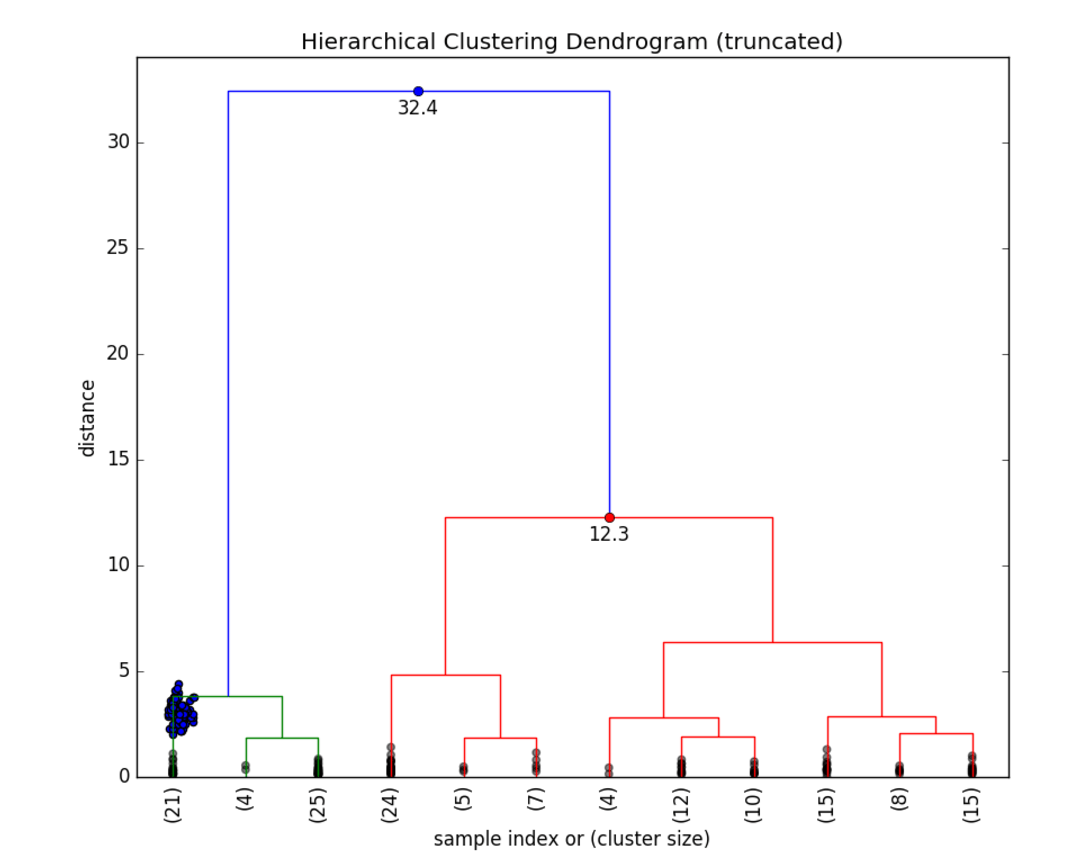Fig 5. HC Clustering Iris dataset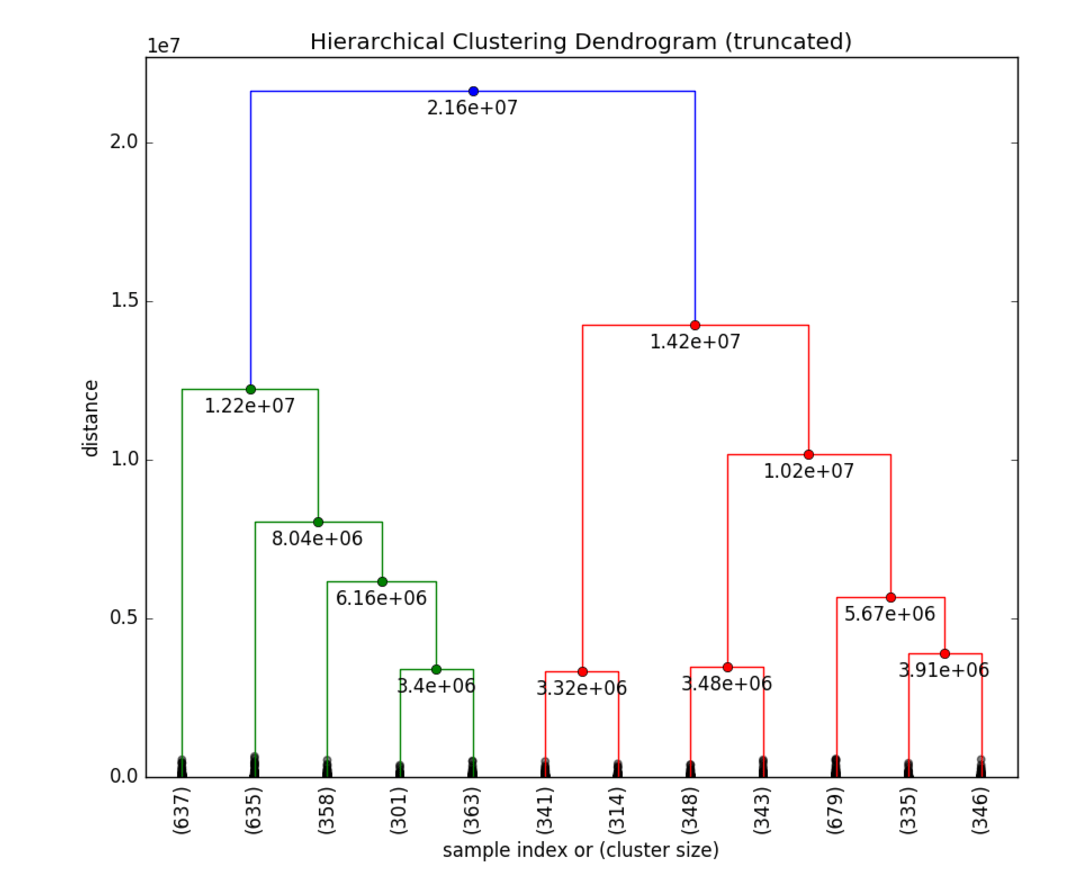Fig 6. HC clustering S1 dataset

Below in the summary of the above clustering experiments.

 Kmeans (sklearn.cluster) AP (sklearn.cluster) HC (scipy.cluster) Birch (sklearn.cluster) Score (Opposite of Sum of distances of samples to their closest cluster center) Silhouette_score Silhouette_score Cophenetic Correlation Coefficient: Silhouette_score Iris dataset, 150, D4 -78.85 0.55 0.52 0.87 0.50 S1 dataset, 5000, D2 -8.92e+12 0.71 * 0.69 0.71

*AP did not work well on S1 dataset (but worked well on iris dataset) however there are some other optional parameters that can be used to resolve this. Probably need to be adjust preference parameter. Currently the tool does not allow change it.

From documentation  Preference is parameter that can be array-like, shape (n_samples,) or float, and is optional. Preferences for each point – points with larger values of preferences are more likely to be chosen as exemplars. The number of exemplars, ie of clusters, is influenced by the input preferences value. If the preferences are not passed as arguments, they will be set to the median of the input similarities.

ML Sandbox
The above tool was used for clustering data. You need just select algorithm, enter your data and click run. Below are detailed instructions for clustering.
How to use the ML Sandbox
1. Open URL: ML Sandbox
2. Select Clustering method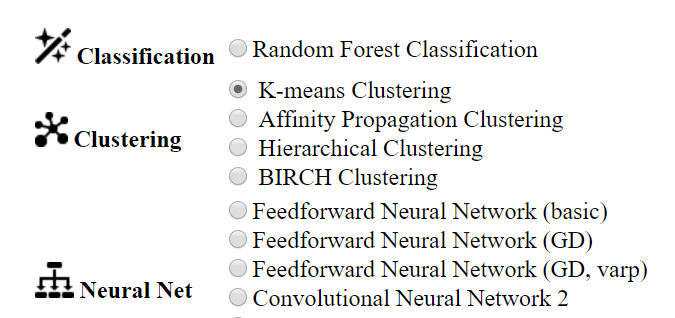3. Enter data (you can use default small dataset or copy and paste your dataset or dataset from other sites like iris, S1 see links in the references section)
4. Click Run Now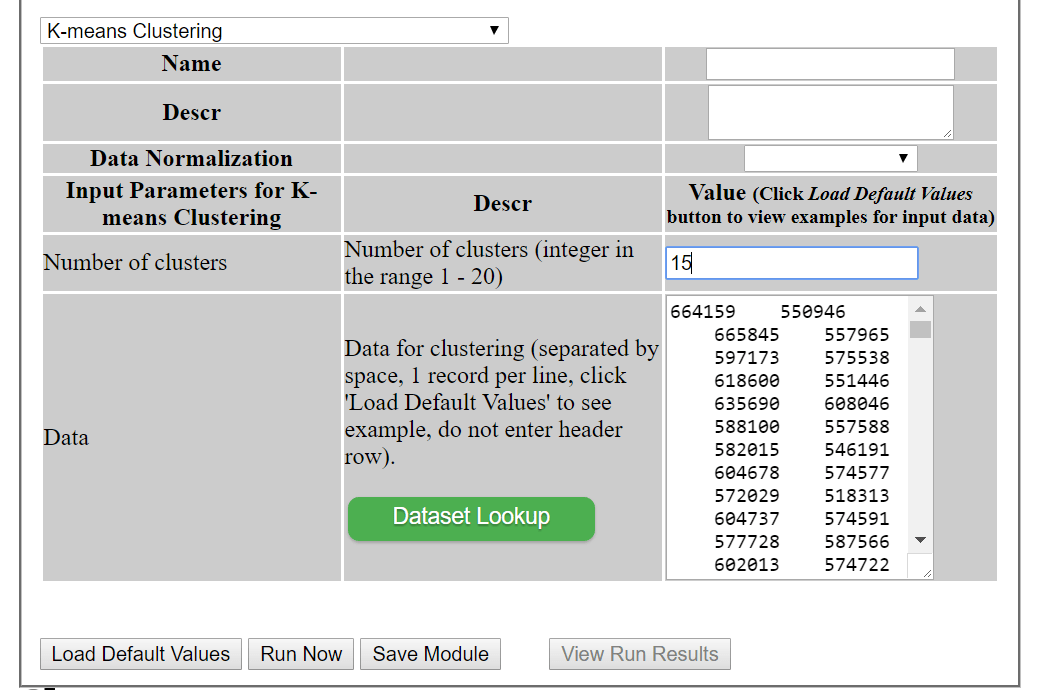5. Click View Run Results
6. If you do not see results, click refresh button at top left corner. Depending on data set and algorithm you might need wait for a minute or two and click refresh.Conclusion
We looked at different clustering methods, metrics performance and visualization of clustering results for different datasets. All of this can be done within online tool ML Sandbox Feel free to play with this tool and your data to explore your datasets. Also feel free to provide any feedback or suggestions.